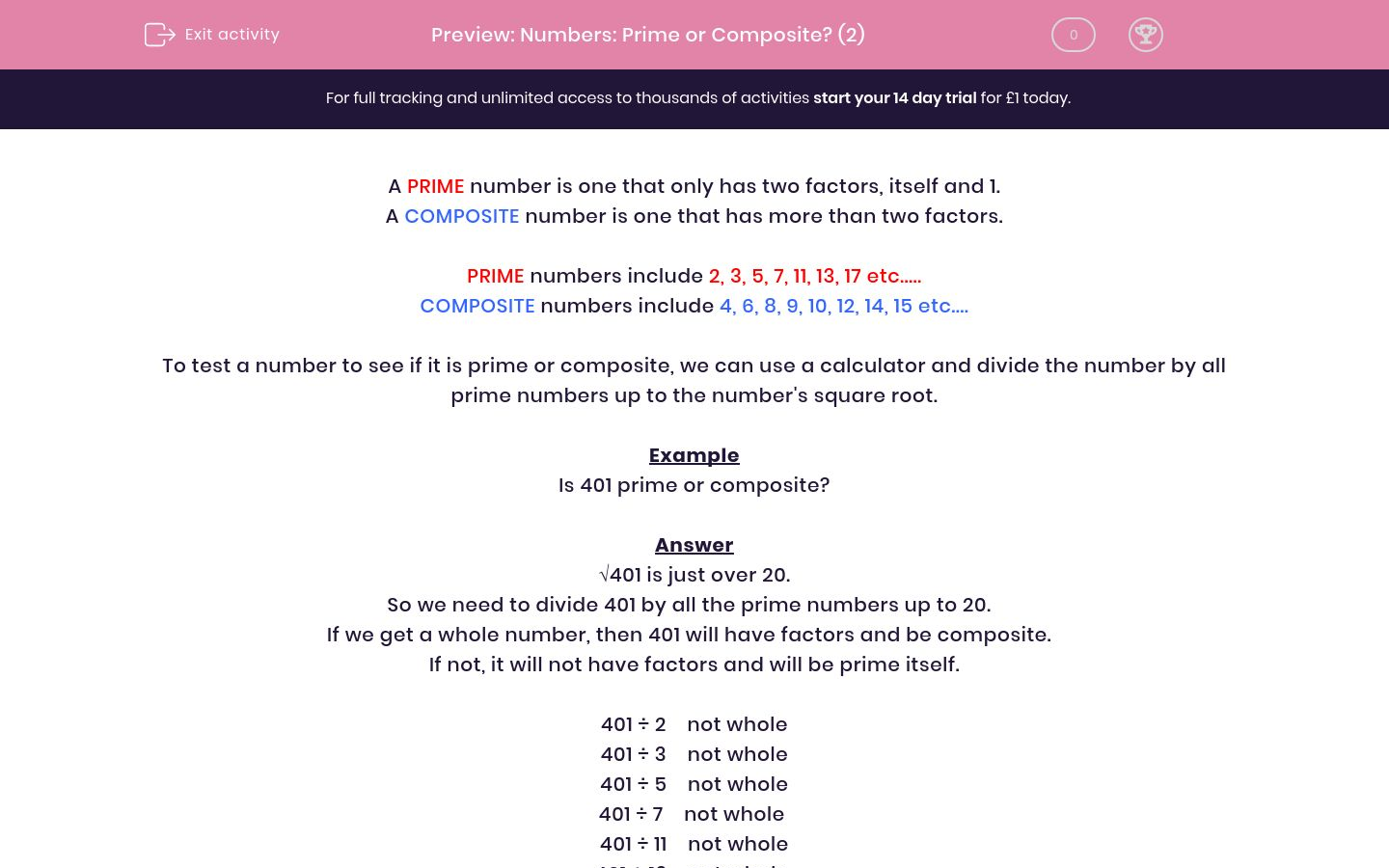# Numbers: Prime or Composite? (2)

In this worksheet, students decide whether or not a given number is prime or composite.Key stage:  KS 2

Curriculum topic:   Maths and Numerical Reasoning

Curriculum subtopic:   Factors and Multiples

Difficulty level:### QUESTION 1 of 10

A PRIME number is one that only has two factors, itself and 1.

A COMPOSITE number is one that has more than two factors.

PRIME numbers include 2, 3, 5, 7, 11, 13, 17 etc.....

COMPOSITE numbers include 4, 6, 8, 9, 10, 12, 14, 15 etc....

To test a number to see if it is prime or composite, we can use a calculator and divide the number by all prime numbers up to the number's square root.

Example

Is 401 prime or composite?

√401 is just over 20.

So we need to divide 401 by all the prime numbers up to 20.

If we get a whole number, then 401 will have factors and be composite.

If not, it will not have factors and will be prime itself.

401 ÷ 2    not whole

401 ÷ 3    not whole

401 ÷ 5    not whole

401 ÷ 7    not whole

401 ÷ 11    not whole

401 ÷ 13    not whole

401 ÷ 17    not whole

401 ÷ 19    not whole

401 is prime.

Is the following number prime or composite?

267

Composite

Prime

Is the following number prime or composite?

279

Composite

Prime

Is the following number prime or composite?

499

Composite

Prime

Is the following number prime or composite?

649

Composite

Prime

Is the following number prime or composite?

839

Composite

Prime

Is the following number prime or composite?

1051

Composite

Prime

Is the following number prime or composite?

391

Composite

Prime

Is the following number prime or composite?

841

Composite

Prime

Is the following number prime or composite?

1213

Composite

Prime

Is the following number prime or composite?

1147

Composite

Prime

• Question 1

Is the following number prime or composite?

267

Composite
EDDIE SAYS
267 = 3 x 89
• Question 2

Is the following number prime or composite?

279

Composite
EDDIE SAYS
279 = 3 x 93
• Question 3

Is the following number prime or composite?

499

Prime
• Question 4

Is the following number prime or composite?

649

Composite
EDDIE SAYS
649 = 11 x 59
• Question 5

Is the following number prime or composite?

839

Prime
• Question 6

Is the following number prime or composite?

1051

Prime
• Question 7

Is the following number prime or composite?

391

Composite
EDDIE SAYS
391 = 17 x 23
• Question 8

Is the following number prime or composite?

841

Composite
EDDIE SAYS
841 = 29 x 29
• Question 9

Is the following number prime or composite?

1213

Prime
• Question 10

Is the following number prime or composite?

1147

Composite
EDDIE SAYS
1147 = 31 x 37
---- OR ----

Sign up for a £1 trial so you can track and measure your child's progress on this activity.

### What is EdPlace?

We're your National Curriculum aligned online education content provider helping each child succeed in English, maths and science from year 1 to GCSE. With an EdPlace account you’ll be able to track and measure progress, helping each child achieve their best. We build confidence and attainment by personalising each child’s learning at a level that suits them.

Get started